# 11-Plus Exam Illustrations - Maths Quiz - Short and Long Division (Questions)

## Short Division

There are all sorts of methods to divide numbers by but there are none so good as long and short division. Other methods are great for teaching the concepts of sharing and some certainly aid mental calculations but they are not very helpful when speed and accuracy on paper are required. Short division uses the 'bus shelter' shape and involves dealing with each digit one at a time, along with any numbers which have been carried over from the previous digit's calculation. Let's start off with a sum: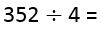Put the question into the following format: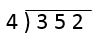There are a lot of children who, bizarrely, then start saying '4 divided by 3' for the next stage when it should be '3 divided by 4'. Even if they are getting the right answer, make sure they say the sum in the right order to avoid confusion. So, it's '3 divided by 4', to which the answer is 0, with 3 left over. That 3 needs to be used so is carried forward to the next digit, the 5, to make 35. We then divide 35 by 4 and put the answer on the top, ABOVE THE 5. If you put it above a different number you may end up getting confused. The answer of course is 8, as 8 x 4 is 32. However, this leaves 3 left over and as we have to use every number, we move it on to the next digit, so 2 becomes 32. Finally, divide 32 by 4 (which is 8) and put this above the 2.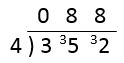There will often be remainders as the figure on the left may not fit into the other number exactly. In this case, either express the remainder as it is or as a fraction of the number on the left. For example, after a sum you may get the answer 43 remainder 3. If the number you were dividing by was 5, the more accurate answer would be 43 and 3/5.

## Long Division

The long division which gave many of our generation nightmares is not that bad as long as you can remember your times tables. Thanks to a move to formalise written methods in recent years, long division is being taught increasingly in year 5 and 6. The concept is the same as short division but, as the numbers are usually greater, we need to record more detail and do work out tougher sums.

Let's see it in action.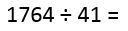Firstly, follow the same 'bus shelter' layout as the short division sum.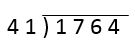Next, follow the technique of short division but write in the answers as you go along. So, divide 1 by 41 - it can't be done. Carry the 1 and make the next number 17. Divide 17 by 41 - again, it can't be done so carry the 17 and put it next to the 6 to make 176. This time you can fit 41 into 176 so work out how many will fit in. Write down the answer above the 6 and write down under the 176 how many 4 x 41 is. 41 fitted into 176 four times, which made 164. Take this from the 176 (12) and bring down the next digit (4), and we have 124 - the next number that we divide 41 into.

The easiest way to work out how many times a large number goes into another is to make notes alongside the sum as you go - 41, 82, 123, 164, 205... If you write your 41 times table (simply add 41 each time) then next time you can refer to it and not have to start from scratch as you'll be dividing by 41 each time. Alternatively, encourage your child to estimate whether it's a high or low number and do some quick calculations, rather than just starting at 1 x 41 etc. If it were a higher number, get them to start at 10 x 41 = 410 and take 41 for 9 x 41.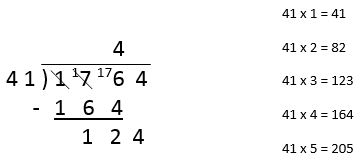This is the same as the short division technique but the sums we do in our heads are formally written down. Now, given that we've made jottings along the side, we can save time and see that 41 fits into 123 three times. Put that 3 on the top as part of the answer and under the 124, put the 123 that we know 41 x 3 is. Find the difference between 124 and 123 and put that number down (1). As there are no more numbers to bring down, this is the remainder.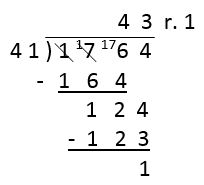Once you get back into the swing of things, it's a lot faster than you remember. Hopefully, your child will remember most of the method and will just need minor assistance to speed up.